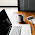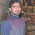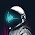# Programming Videos

## Search

### Linear Search Program in C, C++ - Algorithm , Time Complexity

Write a C, C++ program to implement a linear search algorithm.

### Linear Search

Linear search is a simple search algorithm for searching an element in an array. It works by comparing each element of an array. It is the most basic and easiest algorithm in computer science to find an element in a list or an array.

The time complexity of Linear Search is O(n).

For example -

Let's take an array int arr[] = { 2,1,7,5,9}

Suppose we have to search an element 5. Using linear search, We compare 5 with each element of an array. If 5 exists in an array then we return the index.

Binary Search Program in C, C++

### Linear Search Time Complexity

In worst case, the time complexity of linear search is O(n). Worst case occurs, when search element is found at the end of an array.

In terms of time complexity, Binary Search is better than linear search.

C, C++ Interview Questions

Time complexity of sorting algorithm

### Linear Search Program in C++

```#include<iostream>
using namespace std;

int main()
{

int arr, i, size, num, flag = 0;

cout << "Enter the size of an array (Max 50)";
cin >> size;

cout << "Enter the "<< size <<" values in an array ";

for(i = 0; i < size; i++)
{
cin >> arr[i];
}

cout << "Enter the value to be search ";
cin  >> num;

/* Iterate and compare each element of an array
to the value to be searched. */

for(i = 0; i < size; i++)
{
/* If value is found,
then set the flag and break. */
if(arr[i] == num)
{
flag = 1;
break;
}
}

if(flag == 0)

else
cout << " Element is found at position " << (i+1);

return 0;
}  ```
```
```

## Linear Search Program in C

```#include <stdio.h>

int main(void) {

int arr, n, num, i, flag = 0;

/* Take the size of an array */
printf ("Enter the size of an array (Max 50)\n");
scanf ("%d", &n);

printf ("Enter elements in an array \n");

for (i = 0; i < n; i++) {

scanf ("%d", &arr[i]);
}

printf ("Enter the element to be searched for \n");
scanf ("%d", &num);

for (i =0; i < n; i++) {

if (arr[i] == num) {

flag = 1;
break;
}
}

if (flag) {

printf ("Element is found at position %d ", i+1);

} else {

}
return 0;
}

```

Output :

Enter the size of an array (Max 50) : 5

Enter elements in an array :  8     9     3      6      5

Enter the element to be searched : 5

Element is found at position 4

Programming questions on Strings

Programming questions on Recursion

Programming questions on Array

1.cout>>a[i] supposed to be cin>>a[i]

1.Thanks i have corrected.

2.even though i put number that wasn't in the array still saying Value found :3 LOL

1.Hi I have checked this program it is giving correct output.

3.Nice program .... producing required output

4.Loved your logic in C++ program. Here's mine ;)

#include
#include

using namespace std;

int main()
{
int array, i, n, check;

cout<<"Array can support up to 10 numbers.";

for(i=0;i<10;i++)
{
cin>>array[i];
}

cout<<"Enter the number you want to search";
cin>>n;

for(i=0;i<10;i++)
{
if (n==a[i])
{

cout<<"The number has been found at:"<<i;
check=n;

}
}

if (!(check==n))
{
cout<<"The number wasn't found, sorry!";
}

getch();
return (0);
}